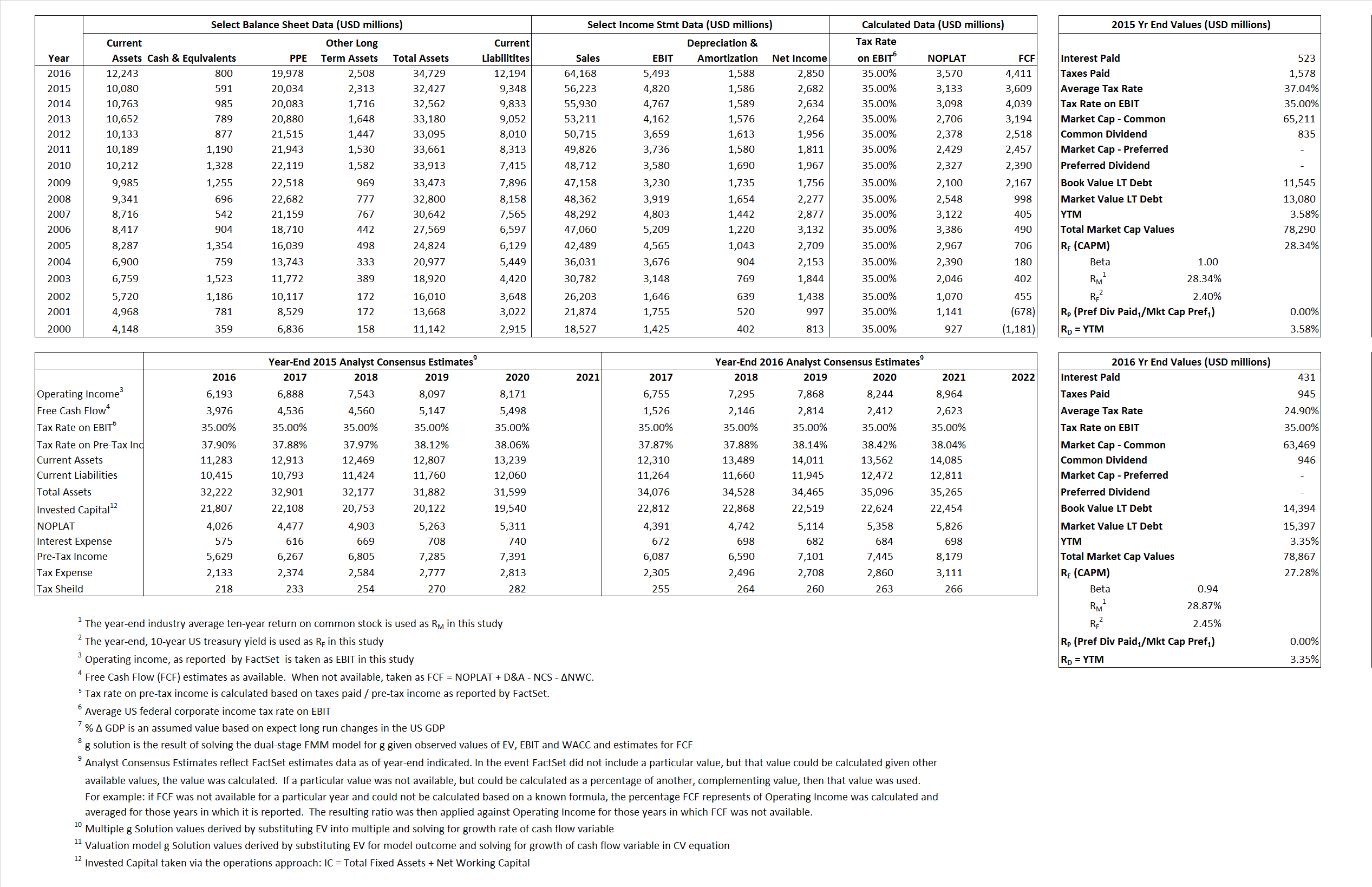# Lowe’s Companies, Inc.

## Analyst Listing

The following analysts provide coverage for the subject firm as of May 2016:

 Broker Analyst Analyst Email Oppenheimer Brian Nagel brian.nagel@opco.com Raymond James Budd Bugatch budd.bugatch@raymondjames.com Jefferies Daniel Binder dbinder@jefferies.com Consumer Edge Research David A. Schick dschick@consumeredgeresearch.com Longbow Research David S. MacGregor dmacgregor@longbowresearch.com Evercore ISI Greg Melich greg.melich@evercoreisi.com Nomura Research Jessica A. Schoen jessica.schoen@nomura.com Telsey Advisory Group Joseph Feldman jfeldman@telseygroup.com SunTrust Robinson Humphrey Keith Hughes keith.hughes@suntrust.com Deutsche Bank Research Mike Baker michael.baker@db.com Piper Jaffray Peter J. Keith peter.j.keith@pjc.com Atlantic Equities Sam Hudson s.hudson@atlantic-equities.com RBC Capital Markets Scot Ciccarelli scot.ciccarelli@rbccm.com Wolfe Research Scott Mushkin smushkin@wolferesearch.com Wedbush Securities Seth Basham seth.basham@wedbush.com BMO Capital Markets Wayne Hood wayne.hood@bmo.com

## Primary Input Data## Derived Input Data

### Equational Form

Net Operating Profit Less Adjusted Taxes NOPLAT  3,133  3,570$NOPLAT\, =\, EBIT\, x\, (1 \,-\, Avg \,\,Tax\,\, Rate\,\, on\,\, EBIT)$
Free Cash Flow FCF 3,609 4,411$FCF\,=NOPLAT\,+\,Non-Cash\,Expenses-\Delta NWC\,-\,NCS$
Tax Shield TS 194 107$TS\,=\,Interest\,\,Paid\,\,x\,\, Avg \,\,Tax\,\,Rate\,\, on\,\, Pre-Tax\,\, Income$
Invested Capital IC 23,079 22,535$IC\,=\,Fixed\,\,Operating\,\,Assets\,\,+\,\,Net\,\, Working\,\, Capital$
Return on Invested Capital ROIC 13.58%  15.84%$ROIC\,=\,\frac { NOPLAT }{ IC }$
Net Investment NetInv 1,936  1,044$NetInv\,=\,{ {IC}_{1}}-{{IC}_{0}}+Depreciation$
Investment Rate IR 61.80% 29.23%$IR\,=\,\frac {NetInv}{NOPLAT}$
Weighted Average Cost of Capital
WACCMarket 23.98% 22.45%$WACC\,=\,\frac { E }{ V } { R }_{ E }\,+\,\frac { P }{ V } { R }_{ P }\,+\,\frac { D }{ V } { R }_{ D }\left( 1- Avg\,\, Tax\,\,Rate\,\,on\,\,Pre-Tax\,\,Income \right)$
WACCBook   7.31%  7.62%
Enterprise value
EVMarket  77,699  78,067$EV\,=\,Market\,\,Cap\,\,Equity\,+\,\,Long\,\,Term\,\,Debt\,-\,Cash$
EVBook   75,956  77,063
Long-Run Growth
g = IR x ROIC
8.39%  4.63% Long-run growth rates of the income variable are used in the Continuing Value portion of the valuation models.
g = %$\Delta$ GDP   2.50%   2.50%
Margin from Operations M   8.57%   8.56%$M\,\,=\,\,\frac{EBIT}{SALES}$
Depreciation/Amortization Rate D  24.76%  22.43%$D\,\,=\,\,\frac{D+A}{EBITDA}$

## Valuation Multiple Outcomes

The outcomes presented in this study are the result of original input data, derived data, and synthesized inputs.

### model g solution

12/31/2015 12/31/2016 12/31/2015 12/31/2016 12/31/2015 12/31/2016

EV/SALES$\frac {EV}{Sales} \,= \,\frac{ROIC\, -\, g}{ROIC\,(WACC\,-\,g)}\,(1\,-\,T)\,(M)$

1.38  1.22  28.38%  25.13%  25.41%  23.22%

EV/EBITDA$\frac {EV}{EBITDA} \,= \,\frac{ROIC\, -\, g}{ROIC\,(WACC\,-\,g)}\,(1\,-\,T)\,(1\,-\,D)$

12.13 11.02 28.38% 25.13% 25.41% 23.22%

EV/NOPLAT$\frac {EV}{NOPLAT} \,= \,\frac{ROIC\, -\, g}{ROIC\,(WACC\,-\,g)}$

24.80 21.86 28.38% 25.13% 25.41% 23.22%

EV/FCFOPS$\frac {EV}{FCF_{OPS}} \,= \,\frac{ROIC\, -\, g}{ROIC\,(WACC\,-\,g)}\,(1\,-\,T)$

21.53 17.70 28.38% 25.13% 25.41% 23.22%

EV/EBIT$\frac {EV}{EBIT} \,= \,\frac{ROIC\, -\, g}{ROIC\,(WACC\,-\,g)}\,(1\,-\,T)$

16.12 14.21 28.38% 25.13% 25.41% 23.22%

EV/IC$\frac {EV}{IC} \,= \,\frac{ROIC\, -\, g}{WACC\,-\,g}$

3.37 3.36 28.38% 25.13% 25.41% 23.22%